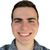# Calculate destination point given distance and bearing from start point

242
2
02-15-2023 05:14 AM
Labels (2)New Contributor

I'm using .Net Maps SDK for a WPF application.
I need to calculate a destination point given a distance and bearing from another start point.

Now I'm using the following method to calculate the destination point.

``````public void demo()
{
MapPoint startPoint = new MapPoint(-7.123456789, 33.123456789);
double bearingDegreesFromNorth = 33;
double distanceMeters = 54321;
// Firts calculate endPoint using startPoint, distance, and bearing.
MapPoint endPoint = CalculateCoordinatesWithOffset(startPoint, distanceMeters, 0, bearingDegreesFromNorth);
// Now with endPoint I calculate again startPoint using the same distance but reverse bearing.
MapPoint startPointAgain = CalculateCoordinatesWithOffset(endPoint, distanceMeters, 0, bearingDegreesFromNorth + 180);

// Result endPoint:{MapPoint[X=-6.80405266951967, Y=33.5330337977028]}
// Result startPointAgain: {MapPoint[X=-7.12195591588525, Y=33.1226432181444]}

}
public static MapPoint CalculateCoordinatesWithOffset(MapPoint mapPoint, double xOffset, double yOffset, double bearing = 0)
{
double distance = Math.Sqrt(Math.Pow(xOffset, 2) + Math.Pow(yOffset, 2));
double latRad = mapPoint.Y * Math.PI / 180.0;
double lonRad = mapPoint.X * Math.PI / 180.0;
double s = distance * Math.PI / (180.0 * 60 * 1852);
// Find the new latitude
// Then the new longitude
double endLon = lonRad + w;
endLat = endLat * 180.0 / Math.PI;
endLon = endLon * 180.0 / Math.PI;
var result = new MapPoint(endLon, endLat);
return result;
}``````

But when I try to calculate the start point using the same distance and the reverse bearing from the destination point, the start point does not coincide.

In the beginning, startPoint value is (-7.123456789, 33.123456789), but when I recalculated the value is [X=-7.12195591588525, Y=33.1226432181444]
Both points are similar, but the last decimals do not coincide.

What is the best way to do that? Is there any way to calculate a point given distance and bearing from the start point and then use the destination point, with the distance and the reverse bearing (bearing +-180º), to calculate the initial point?

1 Solution

Accepted SolutionsbyEsri Contributor

Hello there. I would recommend utilizing the MoveGeodetic method.

``````public static MapPoint CalculateCoord(MapPoint mapPoint, double distance, GeodeticCurveType curveType, double bearing = 0)
{
return GeometryEngine.MoveGeodetic(new List<MapPoint>() { mapPoint }, distance, LinearUnits.Meters, bearing, AngularUnits.Degrees, curveType);
}``````

The mapPoint parameter would need a spatial reference.

In terms of precision - your bearing changes over long distance. So it isn't as simple as bearing + 180 to go from end point to start point.

2 RepliesbyEsri Contributor

Hello there. I would recommend utilizing the MoveGeodetic method.

``````public static MapPoint CalculateCoord(MapPoint mapPoint, double distance, GeodeticCurveType curveType, double bearing = 0)
{
return GeometryEngine.MoveGeodetic(new List<MapPoint>() { mapPoint }, distance, LinearUnits.Meters, bearing, AngularUnits.Degrees, curveType);
}``````

The mapPoint parameter would need a spatial reference.

In terms of precision - your bearing changes over long distance. So it isn't as simple as bearing + 180 to go from end point to start point.New Contributor

Thanks @williambohrmann3, that work to me using  GeodeticCurveType.Loxodrome.